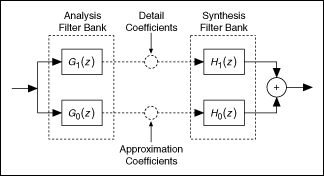Signal Processing with Discrete Wavelets (Advanced Signal Processing Toolkit)

LabVIEW 2014 Advanced Signal Processing Toolkit Help

Edition Date: June 2014

Part Number: 372656C-01

»View Product InfoDownload Help (Windows Only)

Although you can use numerical algorithms to compute continuous wavelet coefficients to analyze a signal, the resulting wavelet coefficients are not invertible. You cannot use those wavelet coefficients to recover the original data samples. For applications that require signal reconstruction, the LabVIEW Wavelet Analysis Tools provide the following discrete wavelet tools:

You can use the discrete wavelet tools to perform signal analysis and signal processing, including multiresolution analysis, denoising, compression, edge detection, peak detection and others.

Selecting an Appropriate Discrete Wavelet

The Wavelet Analysis Tools provide the following commonly used discrete wavelets:

• Orthogonal wavelets—Haar, Daubechies (dbxx), Coiflets (coifx), and Symmlets (symx)
• Biorthogonal wavelets—Biorthogonal (biorx_x), including FBI (bior4_4 (FBI))

x indicates the order of the wavelet. The higher the order, the smoother the wavelet.

Orthogonal wavelets are suitable for applications such as signal and image compression and denoising, because the wavelet transform with orthogonal wavelets possesses the same amount of energy as that contained in the original data samples. The energy-conservative property ensures that the inverse wavelet transform does not enlarge the energy of noise suppressed in the wavelet domain. However, the filters associated with orthogonal wavelets are not linear-phase filters. Linear-phase filters maintain a constant time delay for different frequencies and are necessary in many signal and image feature extraction applications, such as peak detection and image edge detection. Biorthogonal wavelets can be linear phase and are suitable for applications that require linear-phase filters.

You also can use the Wavelet Design Express VI to design a customized wavelet.

Filter Banks

The discrete wavelet transform, the undecimated wavelet transform, and wavelet packet analysis use filter banks, as shown in the following illustration:G1(z) is the analysis highpass filter. G0(z) is the analysis lowpass filter. G1(z) and G0(z) form the analysis filter bank. The output of the analysis highpass filter are the detail coefficients. The output of the analysis lowpass filter are the approximation coefficients. H1(z) is the synthesis highpass filter. H0(z) is the synthesis lowpass filter. H1(z) and H0(z) form the synthesis filter bank.